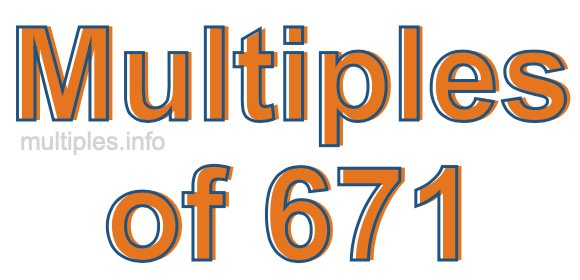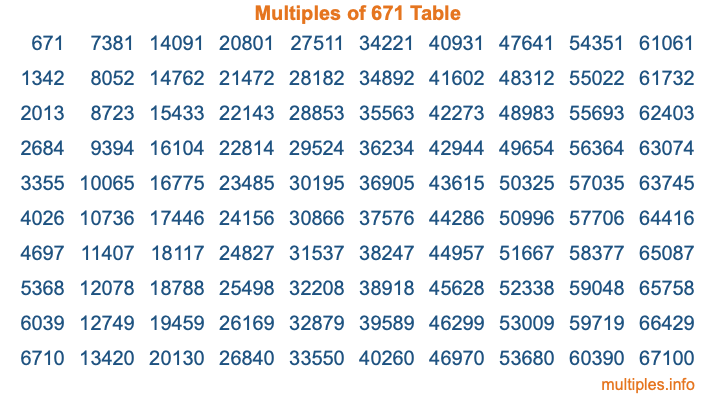Multiples of 671Welcome to the Multiples of 671 page. Here we will first teach you everything you will ever need to know about the multiples of 671, and then give you a study guide summary of everything we taught you to make sure you remember it all. Use this page to look up facts and learn information about the multiples of 671. This page will make you a multiples of six hundred seventy-one expert!

Definition of Multiples of 671
Multiples of 671 are all the numbers that when divided by 671 equal an integer. Each of the multiples of 671 are called a multiple. A multiple of 671 is created by multiplying 671 by an integer.

Therefore, to create a list of multiples of 671, you start with 1 multiplied by 671, then 2 multiplied by 671, then 3 multiplied by 671, and so on for as long as you want. Thus, the list of the first five multiples of 671 is 671, 1342, 2013, 2684, and 3355. To see a larger list of multiples of 671, see the printable image of Multiples of 671 further down on this page. We also have a category where you can choose any nth multiple of 671.

Multiples of 671 Checker
The Multiples of 671 Checker below checks to see if any number of your choice is a multiple of 671. In other words, it checks to see if there is any number (integer) that when multiplied by 671 will equal your number. To do that, we divide your number by 671. If the the quotient is an integer, then your number is a multiple of 671.

Is  a multiple of 671?

Least Common Multiple of 671 and ...
A Least Common Multiple (LCM) is the lowest multiple that two or more numbers have in common. This is also called the smallest common multiple or lowest common multiple and is useful to know when you are adding our subtracting fractions. Enter one or more numbers below (671 is already entered) to find the LCM.

Check out our LCM Calculator if you need more details about the Least Common Multiple or if you need the LCM for different numbers for adding and subtraction fractions.

nth Multiple of 671
As we stated above, 671 is the first multiple of 671, 1342 is the second multiple of 671, 2013 is the third multiple of 671, and so on. Enter a number below to find the nth multiple of 671.

th multiple of 671

Multiples of 671 vs Factors of 671
671 is a multiple of 671 and a factor of 671, but that is where the similarities end. All postive multiples of 671 are 671 or greater than 671. All positive factors of 671 are 671 or less than 671.

Below is the beginning list of multiples of 671 and the factors of 671 so you can compare:

Multiples of 671: 671, 1342, 2013, 2684, 3355, etc.

Factors of 671: 1, 11, 61, 671

As you can see, the multiples of 671 are all the numbers that you can divide by 671 to get a whole number. The factors of 671, on the other hand, are all the whole numbers that you can multiply by another whole number to get 671.

It's also interesting to note that if a number (x) is a factor of 671, then 671 will also be a multiple of that number (x).

Multiples of 671 vs Divisors of 671
The divisors of 671 are all the integers that 671 can be divided by evenly. Below is a list of the divisors of 671.

Divisors of 671: 1, 11, 61, 671

The interesting thing to note here is that if you take any multiple of 671 and divide it by a divisor of 671, you will see that the quotient is an integer.

Multiples of 671 Table
Below is an image of the first 100 multiples of 671 in a table. The table is in chronological order, column by column. The first column has the first ten multiples of 671, the second column has the next ten multiples of 671, and so on.The Multiples of 671 Table is also referred to as the 671 Times Table or Times Table of 671. You are welcome to print out our table for your studies.

Negative Multiples of 671
Although not often discussed or needed in math, it is worth mentioning that you can make a list of negative multiples of 671 by multiplying 671 by -1, then by -2, then by -3, and so on, to get the following list of negative multiples of 671:

-671, -1342, -2013, -2684, -3355, etc.

Multiples of 671 Summary
Below is a summary of important Multiples of 671 facts that we have discussed on this page. To retain the knowledge on this page, we recommend that you read through the summary and explain to yourself or a study partner why they hold true.

There are an infinite number of multiples of 671.

A multiple of 671 divided by 671 will equal a whole number.

671 divided by a factor of 671 equals a divisor of 671.

The nth multiple of 671 is n times 671.

The largest factor of 671 is equal to the first positive multiple of 671.

671 is a multiple of every factor of 671.

671 is a multiple of 671.

A multiple of 671 divided by a divisor of 671 equals an integer.

671 divided by a divisor of 671 equals a factor of 671.

Any integer times 671 will equal a multiple of 671.

Multiples of a Number
Here you can get the multiples of another number, all with the same attention to detail as we did for multiples of 671 on this page.

Multiples of
Multiples of 672
Did you find our page about multiples of six hundred seventy-one educational? Do you want more knowledge? Check out the multiples of the next number on our list!